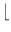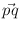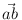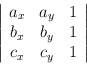Next: Segments ( segment ) Up: Basic Data Types for Previous: Basic Data Types for   Contents   Index

# Points ( point )

Definition

An instance of the data type point is a point in the two-dimensional plane R2. We use (x, y) to denote a point with first (or x-) coordinate x and second (or y-) coordinate y.

#include < LEDA/geo/point.h >

Types

 point::coord_type the coordinate type (double). point::point_type the point type (point).

Creation

 point p introduces a variable p of type point initialized to the point (0, 0). point p(double x, double y) introduces a variable p of type point initialized to the point (x, y). point p(vector v) introduces a variable p of type point initialized to the point (v, v). Precondition: v.dim() = 2. point p(const point& p, int prec) introduces a variable p of type point initialized to the point with coordinates (P*x/P,P*x/P), where p = (x, y) and P = 2prec. If prec is non-positive, the new point has coordinates x and y.

Operations

 double p.xcoord() returns the first coordinate of p. double p.ycoord() returns the second coordinate of p. vector p.to_vector() returns the vector. int p.orientation(const point& q, const point& r) returns orientation(p, q, r) (see below). double p.area(const point& q, const point& r) returns area(p, q, r) (see below). double p.sqr_dist(const point& q) returns the square of the Euclidean distance between p and q. int p.cmp_dist(const point& q, const point& r) returns compare(p.sqrdist(q), p.sqrdist(r)). double p.xdist(const point& q) returns the horizontal distance between p and q. double p.ydist(const point& q) returns the vertical distance between p and q. double p.distance(const point& q) returns the Euclidean distance between p and q. double p.distance() returns the Euclidean distance between p and (0, 0). double p.angle(const point& q, const point& r) returns the angle betweenand. point p.translate_by_angle(double alpha, double d) returns p translated in direction alpha by distance d. The direction is given by its angle with a right oriented horizontal ray. point p.translate(double dx, double dy) returns p translated by vector (dx, dy). point p.translate(const vector& v) returns p+ v, i.e., p translated by vector v. Precondition v.dim() = 2. point p + const vector& v returns p translated by vector v. point p - const vector& v returns p translated by vector - v. point p.rotate(const point& q, double a) returns p rotated about q by angle a. point p.rotate(double a) returns p.rotate( point(0, 0), a). point p.rotate90(const point& q, int i=1) returns p rotated about q by an angle of i x 90 degrees. If i > 0 the rotation is counter-clockwise otherwise it is clockwise. point p.rotate90(int i=1) returns p.rotate90( point(0, 0), i). point p.reflect(const point& q, const point& r) returns p reflected across the straight line passing through q and r. point p.reflect(const point& q) returns p reflected across point q. vector p - const point& q returns the difference vector of the coordinates.

Non-Member Functions

 int cmp_distances(const point& p1, const point& p2, const point& p3, const point& p4) compares the distances (p1,p2) and (p3,p4). Returns +1 (-1) if distance (p1,p2) is larger (smaller) than distance (p3,p4), otherwise 0. point center(const point& a, const point& b) returns the center of a and b, i.e. a +/2. point midpoint(const point& a, const point& b) returns the center of a and b. int orientation(const point& a, const point& b, const point& c) computes the orientation of points a, b, and c as the sign of the determinanti.e., it returns +1 if point c lies left of the directed line through a and b, 0 if a,b, and c are collinear, and -1 otherwise. int cmp_signed_dist(const point& a, const point& b, const point& c, const point& d) compares (signed) distances of c and d to the straight line passing through a and b (directed from a to b). Returns +1 (-1) if c has larger (smaller) distance than d and 0 if distances are equal. double area(const point& a, const point& b, const point& c) computes the signed area of the triangle determined by a,b,c, positive if orientation(a, b, c) > 0 and negative otherwise. bool collinear(const point& a, const point& b, const point& c) returns true if points a, b, c are collinear, i.e., orientation(a, b, c) = 0, and false otherwise. bool right_turn(const point& a, const point& b, const point& c) returns true if points a, b, c form a righ turn, i.e., orientation(a, b, c) < 0, and false otherwise. bool left_turn(const point& a, const point& b, const point& c) returns true if points a, b, c form a left turn, i.e., orientation(a, b, c) > 0, and false otherwise. int side_of_halfspace(const point& a, const point& b, const point& c) returns the sign of the scalar product (b - a)*(c - a). If ba this amounts to: Let h be the open halfspace orthogonal to the vector b - a, containing b, and having a in its boundary. Returns +1 if c is contained in h, returns 0 is c lies on the the boundary of h, and returns -1 is c is contained in the interior of the complement of h. int side_of_circle(const point& a, const point& b, const point& c, const point& d) returns +1 if point d lies left of the directed circle through points a, b, and c, 0 if a,b,c,and d are cocircular, and -1 otherwise. bool inside_circle(const point& a, const point& b, const point& c, const point& d) returns true if point d lies in the interior of the circle through points a, b, and c, and false otherwise. bool outside_circle(const point& a, const point& b, const point& c, const point& d) returns true if point d lies outside of the circle through points a, b, and c, and false otherwise. bool on_circle(const point& a, const point& b, const point& c, const point& d) returns true if points a, b, c, and d are cocircular. bool cocircular(const point& a, const point& b, const point& c, const point& d) returns true if points a, b, c, and d are cocircular. int compare_by_angle(const point& a, const point& b, const point& c, const point& d) compares vectors b-a and d-c by angle (more efficient than calling compare_by_angle(b-a,d-x) on vectors). bool affinely_independent(const array& A) decides whether the points in A are affinely independent. bool contained_in_simplex(const array& A, const point& p) determines whether p is contained in the simplex spanned by the points in A. A may consist of up to 3 points. Precondition The points in A are affinely independent. bool contained_in_affine_hull(const array& A, const point& p) determines whether p is contained in the affine hull of the points in A.Next: Segments ( segment ) Up: Basic Data Types for Previous: Basic Data Types for   Contents   Index